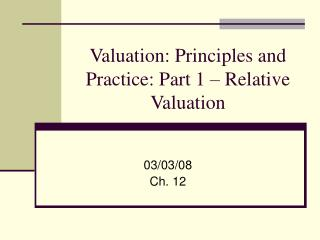# Valuation: Principles and Practice: Part 1 – Relative Valuation - PowerPoint PPT PresentationDownload PresentationValuation: Principles and Practice: Part 1 – Relative Valuation

Valuation: Principles and Practice: Part 1 – Relative ValuationDownload Presentation## Valuation: Principles and Practice: Part 1 – Relative Valuation

- - - - - - - - - - - - - - - - - - - - - - - - - - - E N D - - - - - - - - - - - - - - - - - - - - - - - - - - -
##### Presentation Transcript

1. Valuation: Principles and Practice: Part 1 – Relative Valuation 03/03/08 Ch. 12

2. Valuation techniques • Relative valuation • the value of an asset is derived from the pricing of 'comparable' assets, standardized using a common variable such as earnings, book value or revenues. • Discounted cash flow valuation • The value of an asset is the discounted expected cash flows on that asset at a rate that reflects its riskiness. • Residual Income valuation • The value of an asset is based on the discounted expected difference between net income and its associated cost of equity.

3. Relative valuation • The value of the firm is determined as: Comparable multiple * Firm-specific denominator value where the denominator value can be earnings, book value, sales, etc. • A firm is considered over-valued (under-valued) if the calculated price (or multiple) is greater (less) than the current market price (comparable firm multiple) • Assumptions: • Comparable firms, on average, are fairly valued • Comparable firms have similar fundamental characteristics to the firm being valued.

4. Relative valuation • Examples of relative valuation multiples • Price/Earnings (P/E) • Earnings calculations should exclude all transitory components • Price/Book (P/BV) • Book value of equity is total shareholders equity – preferred stock • Price/Sales (P/S) • Enterprise Value/EBITDA • Enterprise Value = Mkt Cap + Debt – Cash • EBITDA = Earnings before Interest Taxes Depreciation and Amortization

5. Advantages and drawbacks of P/E • Advantages: • Earnings power is the chief driver of investment value • Main focus of security analysts • The P/E is widely recognized and used by investors • Drawbacks • If earnings are negative, P/E does not make economic sense • Reported P/Es may include earnings that are transitory • Earnings can be distorted by management • Assumption: • Required rate of return, retention ratio and growth rates are similar among comparable firms

6. Advantages and drawbacks of P/BV • Advantages • Since book value is a cumulative balance sheet amount, it is generally positive • BV is more stable than EPS, therefore P/BV may be more meaningful when EPS is abnormally low or high • P/BV is particularly appropriate for companies with primarily liquid assets (financial institutions) • Disadvantages • Differences in asset age among companies may make comparing companies difficult • Assumption: • Required rate of return, return on equity, retention ratio and growth rates are similar among comparable firms

7. Advantages and drawbacks of P/S • Advantages • Sales are generally less subject to distortion or manipulation • Sales are positive even when EPS is negative • Sales are more stable than EPS, therefore P/S may be more meaningful when EPS is abnormally low or high • Disadvantages • High growth in sales may not translate to operating profitability • P/S does not reflect differences in cost structure • Assumption: • Required rate of return, profit margin, retention ratio and growth rates are similar among comparable firms

8. Advantages and drawbacks of EV/EBITDA • Advantages • This represents a valuation indicator for the overall company and not just equity. • It is more appropriate for comparing companies that have different capital structures since EBITDA is a pre-interest measure of earnings. • Appropriate for valuing companies with large debt burden: while earnings might be negative, EBIT is likely to be positive. • Disadvantages • Differences in capital investment is not considered. • Assumption: • Required rate of return, growth rates, working capital needs, capital expenditures and depreciation are similar among comparable firms

9. Benchmarks for comparison • Peer companies • Constituent companies are typically similar in their business mix • Industry or sector • Usually provides a larger group of comparables therefore estimates are not as effected by outliers • Overall market • Own historical • This benchmark assumes that the firm will regress to historical average levels • Considerations: market efficiency, historical trends, comparable assumptions

10. Leading and trailing P/E • Trailing (or current) P/Es is calculated using the firm’s current market price and the four most recent quarters’ EPS. • Leading P/Es is calculated using the firm’s current market price and next year’s expected earnings.

11. PEG Ratio • “I don’t buy stocks with P/E’s over 30. To our Foolish ear, that sounds identical to: I don't buy hydrogenated milk; I am born in May.” Motley Fool • When comparable firm P/Es are used to calculate the value of a firm, the assumption is that the firm has characteristics that are similar to that of the average comparable firm. • However, differences may exist. For example, a higher P/E for a particular firm may be justified because the firm has higher growth.

12. PEG Ratio • The Price/Earnings-to-Growth (PEG) accounts for differences in the growth in earnings between companies. • PEG is calculated as: P/E divided by expected earnings growth (%). • "The P/E ratio of any company that's fairly priced will equal its growth rate." Peter Lynch